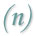One the most commonly used equations governing Open Channel Flow is known as the Mannings’s Equation. It was introduced by the Irish Engineer Robert Manning in 1889 as an alternative to the Chezy Equation. The Mannings equation is an empirical equation that applies to uniform flow in open channels and is a function of the channel velocity, flow area and channel slope.Click here to view an interactive demo of Manning's Equation

Manning’s Equation:Where:

Q = Flow Rate, (ft3/s)

v = Velocity, (ft/s)

A = Flow Area, (ft2)

n = Manning’s Roughness Coefficient